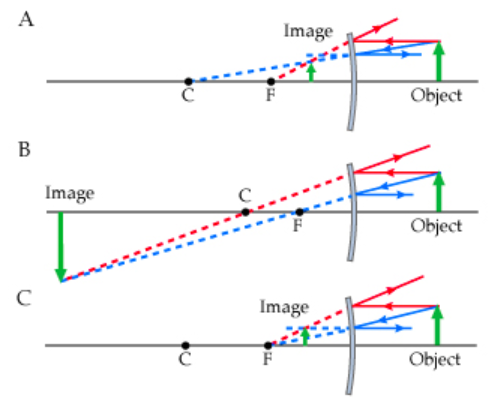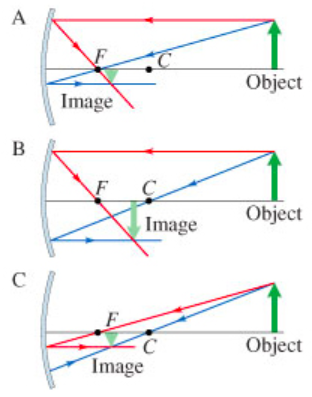# Problem: A candle is placed in front of a convex mirror.Part A. Consider the following diagrams noting that the scale is different between diagrams. In these diagrams, C and F represent the center of curvature and the focal point of the convex mirror, respectively. The image formed by the mirror is obtained using the ray tracing technique. Which diagram(s) are correct? Type A if you think that only diagram A is correct, type AB if you think that only diagrams A and B are correct, and so on.Part B. If the radius of curvature of the convex mirror is 50.0 cm, at what distance d0 from the mirror must the candle be placed so that its image is formed 20 cm from the mirror? Express your answer in centimeters. Part C. Consider the following diagrams, where now C and F represent, respectively, the center of curvature and the focal point of the concave mirror. Again, the image formed by the mirror is found by ray tracing. Choose the correct diagram(s). Type A if you think that only diagram A is correct, type AB if you think that only diagrams A and B are correct, and so on.Part D. Assuming that the candle remains at a distance d0 from the mirror (the same distance found in Part B), at what distance di from the mirror will its image form now? Express your answer in centimeters.

###### FREE Expert Solution

Part A

To draw ray diagrams for convex mirrors, draw two of the following lines:

94% (144 ratings)###### Problem Details

A candle is placed in front of a convex mirror.

Part A. Consider the following diagrams noting that the scale is different between diagrams. In these diagrams, C and F represent the center of curvature and the focal point of the convex mirror, respectively. The image formed by the mirror is obtained using the ray tracing technique. Which diagram(s) are correct? Type A if you think that only diagram A is correct, type AB if you think that only diagrams A and B are correct, and so on.Part B. If the radius of curvature of the convex mirror is 50.0 cm, at what distance d0 from the mirror must the candle be placed so that its image is formed 20 cm from the mirror? Express your answer in centimeters.

Part C. Consider the following diagrams, where now C and F represent, respectively, the center of curvature and the focal point of the concave mirror. Again, the image formed by the mirror is found by ray tracing. Choose the correct diagram(s). Type A if you think that only diagram A is correct, type AB if you think that only diagrams A and B are correct, and so on.Part D. Assuming that the candle remains at a distance d0 from the mirror (the same distance found in Part B), at what distance di from the mirror will its image form now? Express your answer in centimeters.